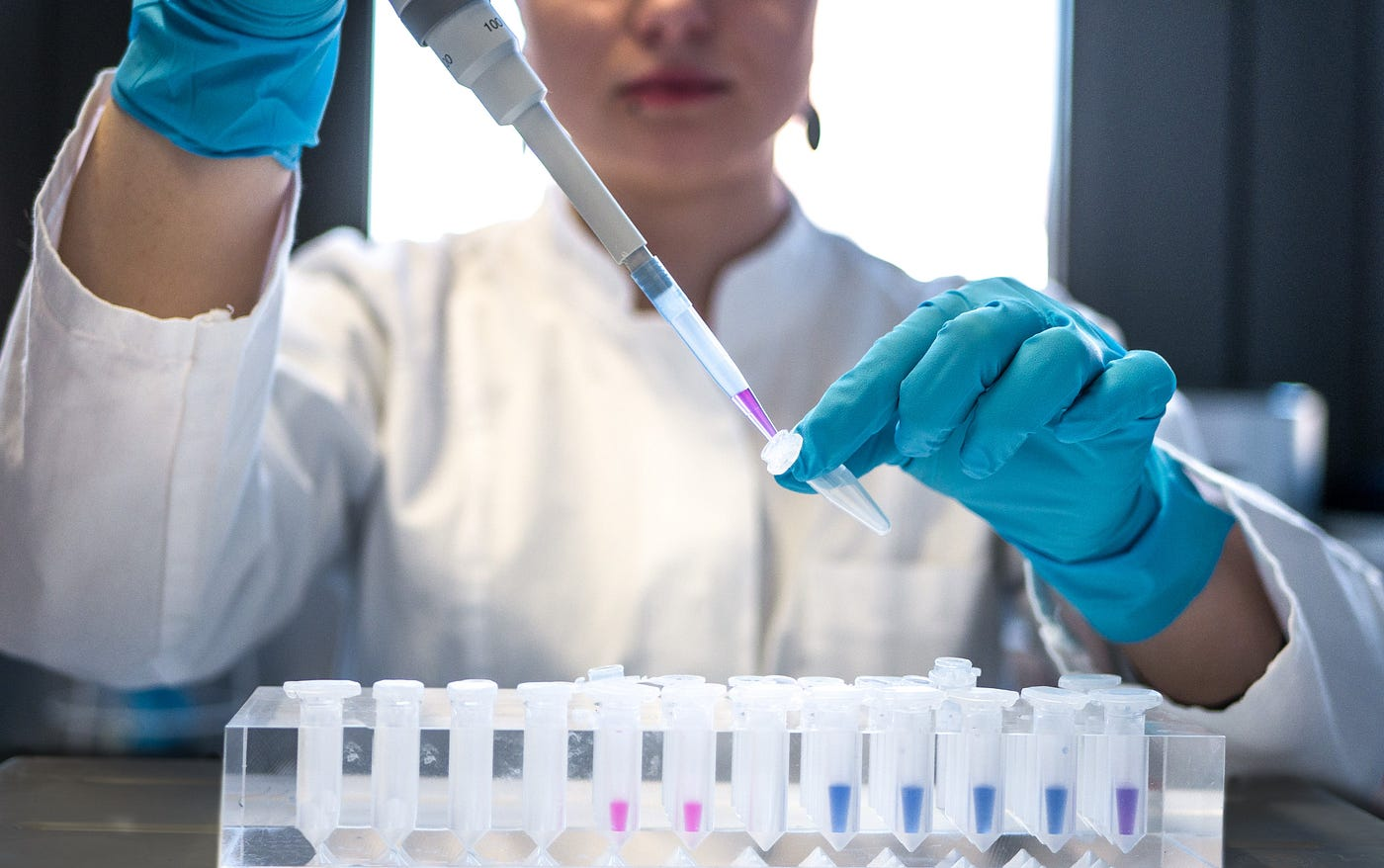# Jarque-Bera Test with Python. Having your data follow a normal… | by John DeJesus | Aug, 2022

Having your data follow a normal distribution is great since it would allow you to take advantage of all the benefits a normal distribution provides. But how do we know our data is following a normal distribution? That is where the Jarque-Bera Test comes in. In this article, we will learn about the Jarque-Bera Test, how we can apply it to our data in Python with Scipy, some quick practice, and create the test statistic equation from scratch.Photo by Julia Koblitz on Unsplash

The Jarque-Bera Test is a test to determine if a set of data values follows the normal distribution based on the data’s skewness and kurtosis. The test statistic equation incorporating skewness and kurtosis is:Jarque Bera Test Statistic Equation. Made by Author on https://latex.codecogs.com/eqneditor/editor.php

Where n = the number of values for the data. S is the sample skewness (how much the data leans away from the mean) as defined below:Sample Skewness for Jarque Bera Test Statistic Equation. Made by Author on https://latex.codecogs.com/eqneditor/editor.php

K is the sample kurtosis (how thick the tails of the distribution are) as defined below:Sample Kurtosis for Jarque Bera Test Statistic Equation. Made by Author on https://latex.codecogs.com/eqneditor/editor.php

The test statistic result will always be greater than or equal to zero since:

1. The sample skewness in the test statistic equation is always squared, meaning S² is always positive or zero.
2. The sample kurtosis is always positive or zero since the numerator is raised to the 4th power and the denominator is squared.
3. The difference between the sample kurtosis and 3 is squared, meaning this term of the test statistic equation is always positive or zero.
4. The sum of two terms ≥ 0 will also be greater than or equal to zero.

We know if our data follows a normal distribution if the test statistic is close to zero and the p-value is larger than our standard 0.05. The p-value relates to a null hypothesis that the data is following a normal distribution. If the test statistic is large and the p-value is less than 0.05, the data does not follow a normal distribution.

Now that we have the test statistic established, you can pull a data set with numeric features and apply the Jarque-Bera Test to them. For this article, I will use the Popular Baby Names data set from OpenData NYC.

## Data Set Up and Exploration

First, we will load our import our necessary packages and import our data. Notice we are importing the `jarque_bera` function from Scipy. We then inspect the data with `pd.info()`.Results of pandas.info() on Popular Baby Names Data